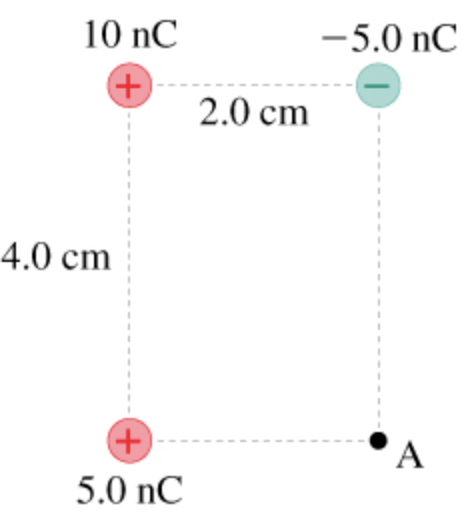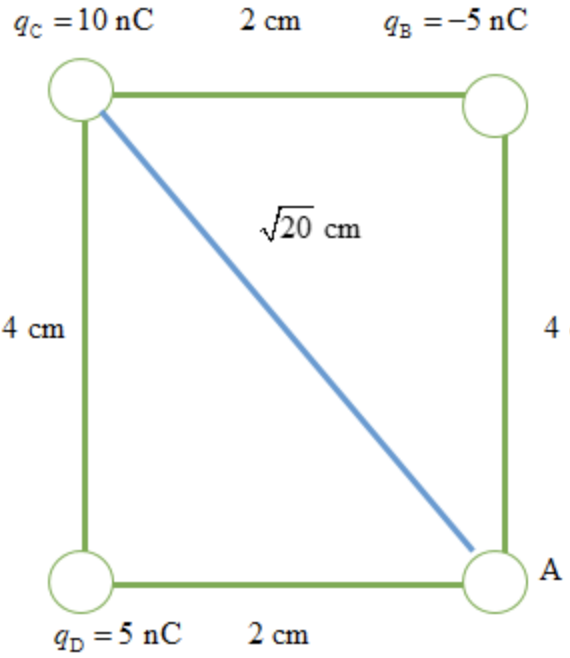In: Physics

# What is the electric potential at point A in the figure?a) What is the electric potential at point A in the figure?

b)What is the potential energy of a proton at point A in the figure?

## Solutions

##### Expert Solution

Concepts and reason

The concepts used to solve the question is the diagram for the given situation, electric potential, and the electric potential energy. At the first draw, the diagram for the situation and then use an electric potential formula to determine the electric potential at point A. At last, calculate the electric potential energy of the proton.

Fundamentals

Electric potential. When a charged particle is placed at some region, if any other particle is brought into this region, it will experience a force. The region around the charged particle is termed as an electric field. When a charged particle is moved against the electric field's force, the charged particle's potential energy increases. Thus, the electric potential is defined as the ratio of the potential energy to the particle's charge. Mathematically the electric potential is defined as, $$V=\frac{k q}{r}$$

Here, $$k$$ is the Coulomb constant, $$q$$ is the charge of the particle, and $$r$$ is the distance of the point charge to the point in space where potential is to be calculated. Electrostatic potential energy. It is defined as the energy required to move the charge from one position to another. It has units of joules. Let us consider a positively charged particle that creates an electric field around it. If another positively charged particle is brought into this region, it will experience a repulsive force from the particle first. If we move the second charged particle against the repulsive force, the work is needed to be done. Thus, the work needed to move this charged particle is termed electrostatic potential energy. Mathematically it is defined as, $$U=q V$$

Here, $$q$$ is the charge of the particle, and $$V$$ is the potential difference.

(a) The diagram for the question is drawn belowThe potential at the point A due to all the charges is, $$V=\frac{k q_{\mathrm{B}}}{\mathrm{AB}}+\frac{k q_{\mathrm{C}}}{\mathrm{AC}}+\frac{k q \mathrm{D}}{\mathrm{AD}} \ldots \ldots$$ (1)

Substitute $$9 \times 10^{9} \mathrm{~N} \cdot \mathrm{m}^{2} / \mathrm{C}^{2}$$ for $$k, 5 \mathrm{n} \mathrm{C}$$ for $$q_{\mathrm{D}},-5 \mathrm{n} \mathrm{C}$$ for $$q_{\mathrm{B}}, 10 \mathrm{n} \mathrm{C}$$ for $$q_{\mathrm{C}}, \sqrt{20} \mathrm{~cm}$$ for $$\mathrm{BC}, 2 \mathrm{~cm}$$ for $$\mathrm{AD}$$

and $$4 \mathrm{~cm}$$ for $$\mathrm{AB}$$ in the equation (1)

$$V=\frac{k\left(5 \mathrm{nC}\left(\frac{10^{-9} \mathrm{C}}{\ln \mathrm{C}}\right)\right)}{2 \mathrm{~cm}\left(\frac{10^{-2} \mathrm{~m}}{1 \mathrm{~cm}}\right)}+\frac{k\left(10 \mathrm{nC}\left(\frac{10^{-9} \mathrm{C}}{1 \mathrm{nC}}\right)\right)}{\sqrt{20 \mathrm{~cm}}\left(\frac{10^{-2} \mathrm{~m}}{1 \mathrm{~cm}}\right)}+\frac{k\left(-5 \mathrm{n} \mathrm{C}\left(\frac{10^{-9} \mathrm{C}}{\ln \mathrm{C}}\right)\right)}{4 \mathrm{~cm}\left(\frac{10^{-2} \mathrm{~m}}{1 \mathrm{~cm}}\right)}$$

$$=\left(9 \times 10^{9} \mathrm{~N} \cdot \mathrm{m}^{2} / \mathrm{C}^{2}\right)\left(5 \mathrm{nC}\left(\frac{10^{-9} \mathrm{C}}{1 \mathrm{nC}}\right)\right)(25)+\frac{\left(9 \times 10^{9} \mathrm{~N} \cdot \mathrm{m}^{2} / \mathrm{C}^{2}\right)\left(10 \mathrm{nC}\left(\frac{10^{-9} \mathrm{C}}{\ln \mathrm{C}}\right)\right)}{4.472 \mathrm{~cm}\left(\frac{10^{-2} \mathrm{~m}}{1 \mathrm{~cm}}\right)}$$

$$=1125 \mathrm{~V}+2012.522 \mathrm{~V}$$

The total potential at the point $$\mathrm{A}$$ is, $$V=3137.522 \mathrm{~V}$$

Part a

The potential at point A due to all the charges is $$3137.522 \mathrm{~V}$$

The potential at point A is the sum of the potentials of all the charges. Thus, at point A, the net potential is determined by calculating the potential of each charge.

(b) The electric potential energy of the proton at the point $$\mathrm{A}$$ is, $$U=q_{\mathrm{p}} V \ldots \ldots(1)$$

Here, $$q_{\mathrm{p}}$$ is the charge of the proton. Substitute $$1.6 \times 10^{-19} \mathrm{C}$$ for $$q_{\mathrm{p}}$$ and $$3137.522 \mathrm{~V}$$ for $$V$$ in the equation (1)

$$U=q_{\mathrm{p}} V$$

$$=\left(1.6 \times 10^{-19} \mathrm{C}\right)(3137.522 \mathrm{~V})$$

$$=5.020 \times 10^{-16} \mathrm{~J}$$

Part b The electrostatic potential energy at point $$\mathrm{A}$$ is $$\mathbf{5} . \mathbf{0} \mathbf{2} \mathbf{0} \times \mathbf{1} \mathbf{0}^{-\mathbf{1} \mathbf{6}} \mathbf{J}$$

The electrostatic potential energy is the energy needed to move the proton to point $$\mathrm{A}$$ and it is directly related to the charge of the particle and potential of the charge.